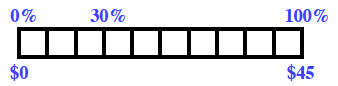### Home > MC1 > Chapter 8 > Lesson 8.2.2 > Problem8-38

8-38.

Tires R Us is having a $30\%$ off sale. Use a percent ruler to find your savings on a tire that regularly costs $45$.

Your percent ruler might look something like this:Using the lesson 4.1.4 Math Notes box as a reference, can you scale the rest of the ruler in order to find the $30\%$ equivalent in dollars? It may help to first find the value of $10\%$.

$30\%$ of $45$ is $13.50$. Remember to show your work!